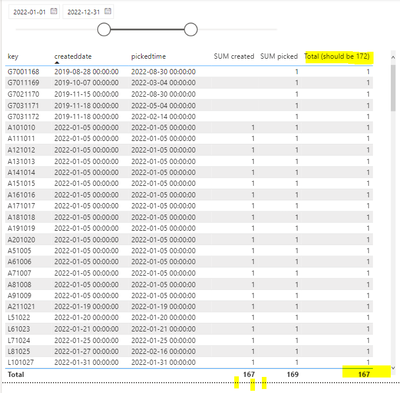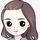cancel
Showing results for
Did you mean:Helper II

## Distinct count of created OR picked within selected range

My problem is that I need the distinct count of a key if the "createddate" is within the filtered OR if the "pickedtime" is within the selected range.

I have a direct relationship between Dim'[Date] and Table'[createddate] and an indirect relationship between Dim'[Date] and Table'[pickedtime]

I have a count of 167 keys with createddate within the range, and 169 keys with pickeddate within the range. One of the records has both createddate and pickedtime within the range, and this should be counted as 1.

I want to count the keys if createddate or pickedtime is within the selected *DimDate[Date] range.

In total there should be 172 distinct keys within the range 2022-01-01-2022-12-31with this logic.

In the table below the "Total" column looks right, as there is a "1" for each value, but the sum is still 167, not 172.

I am using the following measures:

SUM created = DISTINCTCOUNT('Table'[key])
SUM picked = calculate(DISTINCTCOUNT('Table'[key]);USERELATIONSHIP(DimDate[Date];'Table'[pickedtime]))
Total (should be 172) = calculate(If([SUM created]>0;[SUM created];[SUM picked]))

I hope to find a way to enclose the .pbix file here 🙂

Very grateful for help!1 ACCEPTED SOLUTIONCommunity Support

Hi @Goaanna ,

You can create another measure to replace the original measure [Total (should be 172)] and put this new measure onto the visual...

``````Measure =
VAR _tab1 =
VALUES ( 'Table'[key] )
VAR _tab2 =
CALCULATETABLE (
VALUES ( 'Table'[key] ),
USERELATIONSHIP ( DimDate[Date], 'Table'[pickedtime] )
)
VAR _tab =
DISTINCT ( UNION ( _tab1, _tab2 ) )
RETURN
SUMX ( _tab, [Total (should be 172)] )``````Best Regards

Community Support Team _ Rena
If this post helps, then please consider Accept it as the solution to help the other members find it more quickly.
5 REPLIES 5Helper II

### Hi, thank you so much @v-yiruan-msft! That did the trick. I am so grateful after many hours of trying to get my head around this. Thanks again, really really appreciated!!!Super User

Hey @Goaanna ,

why should the amount be 172?

With the last if you just display the measure [SUM created] what is 167. So why would you expect 172?

Also the last CALCULATE doesn't make any sense as you don't change any filter context. Just write:

``Total (should be 172) = If( [SUM created]>0; [SUM created]; [SUM picked] )``

Unfortunately you cannot upload here directly. Maybe share a OneDrive or Dropbox link or use a sharing service like https://www.swisstransfer.com to share your file.

If you need any help please let me know.
If I answered your question I would be happy if you could mark my post as a solution ✔️ and give it a thumbs up 👍

Best regards
Denis

Blog: WhatTheFact.biHelper II

There are 5 keys with picked dates in the interval but null created dates. So the total is 167 keys with created dates + 5 keys with picked dates = total of 172.

(Or 169 with picked dates and 3 with null picked dates but with created dates = 172)

I am unable to achieve this total and still need help.

(Thanks for the calculate tip, was left over from when I was trying with filters. I removed it and of course no change. And thanks, good tip with swiss transfer :-))Helper II

Here is my .pbix file:Community Support

Hi @Goaanna ,

You can create another measure to replace the original measure [Total (should be 172)] and put this new measure onto the visual...

``````Measure =
VAR _tab1 =
VALUES ( 'Table'[key] )
VAR _tab2 =
CALCULATETABLE (
VALUES ( 'Table'[key] ),
USERELATIONSHIP ( DimDate[Date], 'Table'[pickedtime] )
)
VAR _tab =
DISTINCT ( UNION ( _tab1, _tab2 ) )
RETURN
SUMX ( _tab, [Total (should be 172)] )``````Best Regards

Community Support Team _ Rena
If this post helps, then please consider Accept it as the solution to help the other members find it more quickly.